IMPROPER INTEGRALS
Recall the FUNDAMENTAL THEOREM OF CALCULUS :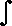b a
f (x) dx   =   F(b) - F(a) where F' = f

Under the simplest interpretation, " a " , " b " , F(a) , and F(b) must be numbers, and the function " f " must be defined and continuous in the interval [a,b]. However, we can often extend this tool to more bizarre situations, such as the following.

A plane region R is called BOUNDED if it is contained within some circle. Triangles, squares, line segments, and circles are all bounded, but lines, parabolas, and hyperbolas are unbounded.

An integral is called IMPROPER if it describes the area of an unbounded region. Thus, the left-hand integral below is "proper" and describes the area of a triangle. The right-hand integral is improper and describes an area (discussed in detail below) which extends indefinitely to the right.

A "proper" integral :   An "improper" integral :b a
(2x + 3) dx1
 1x2
dx

One way to study improper integrals is to temporarily chop off an unbounded part of the area, find the size of the remaining bounded area, and then find the limit as the chop point is extended to include more and more area. If the limit does not exist, the area is said to be infinite ; however, often the limit exists, as for the improper integral above, and that limit becomes the value of the integral. The chop point in our example will be the red number N .

Thus, we write :1
 1x2
dx   =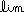NN 1
 1x2
dx = A = AREA UNDER THE GRAPH BELOW

The above limit can be calculated :
=N( -
 1 x
) |
 N 1
=N( -
 1 N
+ 1 ) = -0 + 1 = 1

For the exercises below, moving your mouse across the graph allows you to control the chop number N and the amount of area counted. If you slide your mouse off the bottom edge of the graph, the chosen area will remain visible. When you have selected a chop number N, you may answer the questions below. The shape and scale of the graph below is exaggerated for artistic effect.

 Move the mouse arrow slowly across the graph at the right. THE AMOUNT OF ORANGE AREA WILL CHANGE. To keep a chosen area visible, slide your mouse off the bottom edge of the graph.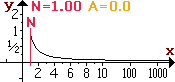Use your mouse to show an orange area with size = 0.5 ; when you are done,
  Use your mouse to show an orange area with size = 0.9 ; when you are done,
  Use your mouse to show an orange area with size = 0.99 ; when you are done,
  Use your mouse to show an orange area with size = 0.999 ; when you are done,
  If the graph had been extended to x=one million, would the orange area exceed 1.0? ;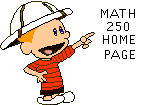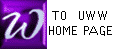This page copyrighted: